#### Howdy, Stranger!

We are about to switch to a new forum software. Until then we have removed the registration on this forum.

#### In this Discussion

edited June 2016

Implemented the shadow mapping technique from this old tutorial (without using any "low level GL") in Processing 3.0.X.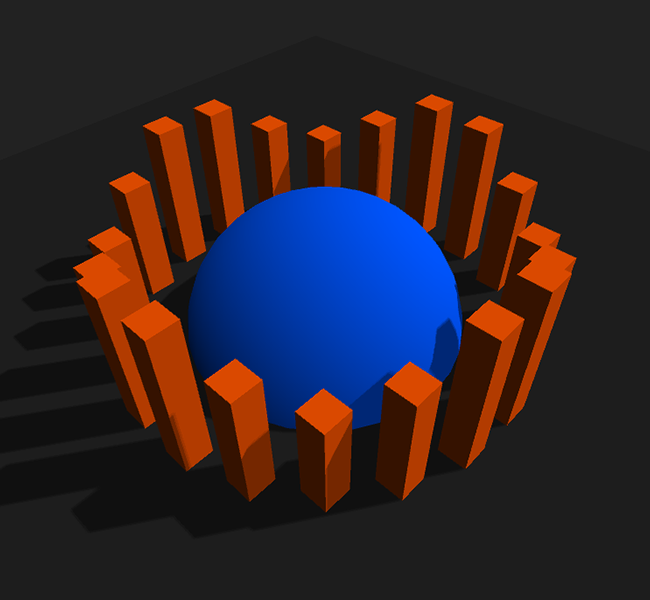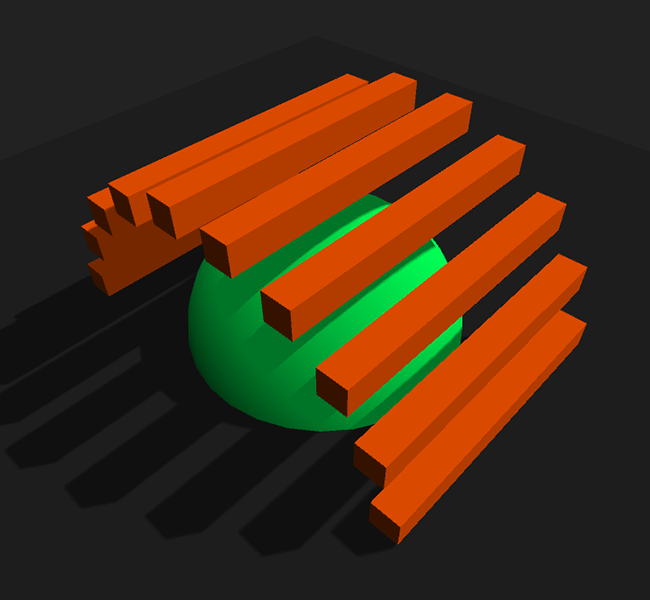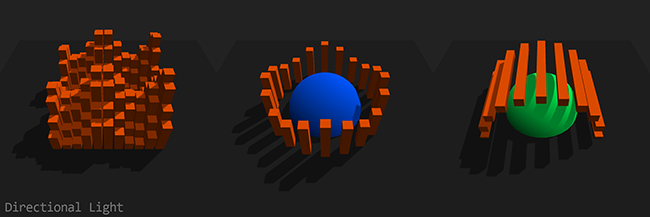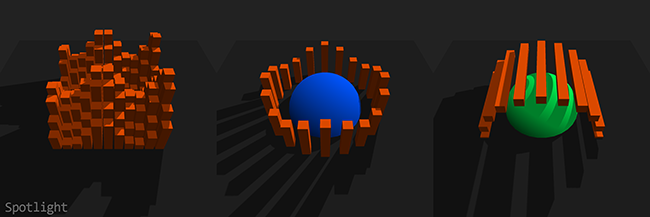Press 1, 2 or 3 to switch between the different demo "landscapes", s for spotlight and d for directional light.

``````import peasy.*;

PVector lightDir = new PVector();
int landscape = 1;

void setup() {
size(800, 800, P3D);
new PeasyCam(this, 300).rotateX(4.0);
initDefaultPass();
}

void draw() {

// Calculate the light direction (actually scaled by negative distance)
float lightAngle = frameCount * 0.002;
lightDir.set(sin(lightAngle) * 160, 160, cos(lightAngle) * 160);

shadowMap.camera(lightDir.x, lightDir.y, lightDir.z, 0, 0, 0, 0, 1, 0);
shadowMap.background(0xffffffff); // Will set the depth to 1.0 (maximum depth)

// Update the shadow transformation matrix and send it, the light

// Render default pass
background(0xff222222);
renderLandscape(g);

// Render light source
pushMatrix();
fill(0xffffffff);
translate(lightDir.x, lightDir.y, lightDir.z);
box(5);
popMatrix();

}

String[] vertSource = {
"uniform mat4 transform;",

"attribute vec4 vertex;",

"void main() {",
"gl_Position = transform * vertex;",
"}"
};
String[] fragSource = {

// In the default shader we won't be able to access the shadowMap's depth anymore,
// just the color, so this function will pack the 16bit depth float into the first
// two 8bit channels of the rgba vector.
"vec4 packDepth(float depth) {",
"float depthFrac = fract(depth * 255.0);",
"return vec4(depth - depthFrac / 255.0, depthFrac, 1.0, 1.0);",
"}",

"void main(void) {",
"gl_FragColor = packDepth(gl_FragCoord.z);",
"}"
};
shadowMap.ortho(-200, 200, -200, 200, 10, 400); // Setup orthogonal view matrix for the directional light
}

public void initDefaultPass() {
String[] vertSource = {
"uniform mat4 transform;",
"uniform mat4 modelview;",
"uniform mat3 normalMatrix;",
"uniform vec3 lightDirection;",

"attribute vec4 vertex;",
"attribute vec4 color;",
"attribute vec3 normal;",

"varying vec4 vertColor;",
"varying float lightIntensity;",

"void main() {",
"vertColor = color;",
"vec4 vertPosition = modelview * vertex;", // Get vertex position in model view space
"vec3 vertNormal = normalize(normalMatrix * normal);", // Get normal direction in model view space
"lightIntensity = 0.5 + dot(-lightDirection, vertNormal) * 0.5;",
"gl_Position = transform * vertex;",
"}"
};
String[] fragSource = {
"#version 120",

// Used a bigger poisson disk kernel than in the tutorial to get smoother results
"const vec2 poissonDisk = vec2[] (",
"vec2(0.95581, -0.18159), vec2(0.50147, -0.35807), vec2(0.69607, 0.35559),",
"vec2(-0.0036825, -0.59150), vec2(0.15930, 0.089750), vec2(-0.65031, 0.058189),",
"vec2(0.11915, 0.78449), vec2(-0.34296, 0.51575), vec2(-0.60380, -0.41527)",
");",

// Unpack the 16bit depth float from the first two 8bit channels of the rgba vector
"float unpackDepth(vec4 color) {",
"return color.r + color.g / 255.0;",
"}",

"varying vec4 vertColor;",
"varying float lightIntensity;",

"void main(void) {",

// Project shadow coords, needed for a perspective light matrix (spotlight)

// Only render shadow if fragment is facing the light
"if(lightIntensity > 0.5) {",
"float visibility = 9.0;",

// I used step() instead of branching, should be much faster this way
"for(int n = 0; n < 9; ++n)",

"gl_FragColor = vec4(vertColor.rgb * min(visibility * 0.05556, lightIntensity), vertColor.a);",
"} else",
"gl_FragColor = vec4(vertColor.rgb * lightIntensity, vertColor.a);",

"}"
};
noStroke();
perspective(60 * DEG_TO_RAD, (float)width / height, 10, 1000);
}

// Bias matrix to move homogeneous shadowCoords into the UV texture space
0.5, 0.0, 0.0, 0.5,
0.0, 0.5, 0.0, 0.5,
0.0, 0.0, 0.5, 0.5,
0.0, 0.0, 0.0, 1.0
);

// Apply project modelview matrix from the shadow pass (light direction)

// Apply the inverted modelview matrix from the default pass to get the original vertex
// positions inside the shader. This is needed because Processing is pre-multiplying
// the vertices by the modelview matrix (for better performance).
PMatrix3D modelviewInv = ((PGraphicsOpenGL)g).modelviewInv;

// Convert column-minor PMatrix to column-major GLMatrix and send it to the shader.
// PShader.set(String, PMatrix3D) doesn't convert the matrix for some reason.
));

// Calculate light direction normal, which is the transpose of the inverse of the
// modelview matrix and send it to the default shader.
float lightNormalX = lightDir.x * modelviewInv.m00 + lightDir.y * modelviewInv.m10 + lightDir.z * modelviewInv.m20;
float lightNormalY = lightDir.x * modelviewInv.m01 + lightDir.y * modelviewInv.m11 + lightDir.z * modelviewInv.m21;
float lightNormalZ = lightDir.x * modelviewInv.m02 + lightDir.y * modelviewInv.m12 + lightDir.z * modelviewInv.m22;
float normalLength = sqrt(lightNormalX * lightNormalX + lightNormalY * lightNormalY + lightNormalZ * lightNormalZ);
defaultShader.set("lightDirection", lightNormalX / -normalLength, lightNormalY / -normalLength, lightNormalZ / -normalLength);

}

public void keyPressed() {
if(key != CODED) {
if(key >= '1' && key <= '3')
landscape = key - '0';
else if(key == 'd') {
} else if(key == 's') {
}
}
}

public void renderLandscape(PGraphics canvas) {
switch(landscape) {
case 1: {
float offset = -frameCount * 0.01;
canvas.fill(0xffff5500);
for(int z = -5; z < 6; ++z)
for(int x = -5; x < 6; ++x) {
canvas.pushMatrix();
canvas.translate(x * 12, sin(offset + x) * 20 + cos(offset + z) * 20, z * 12);
canvas.box(10, 100, 10);
canvas.popMatrix();
}
} break;
case 2: {
float angle = -frameCount * 0.0015, rotation = TWO_PI / 20;
canvas.fill(0xffff5500);
for(int n = 0; n < 20; ++n, angle += rotation) {
canvas.pushMatrix();
canvas.translate(sin(angle) * 70, cos(angle * 4) * 10, cos(angle) * 70);
canvas.box(10, 100, 10);
canvas.popMatrix();
}
canvas.fill(0xff0055ff);
canvas.sphere(50);
} break;
case 3: {
float angle = -frameCount * 0.0015, rotation = TWO_PI / 20;
canvas.fill(0xffff5500);
for(int n = 0; n < 20; ++n, angle += rotation) {
canvas.pushMatrix();
canvas.translate(sin(angle) * 70, cos(angle) * 70, 0);
canvas.box(10, 10, 100);
canvas.popMatrix();
}
canvas.fill(0xff00ff55);
canvas.sphere(50);
}
}
canvas.fill(0xff222222);
canvas.box(360, 5, 360);
}
``````

I mostly commented the Processing part of the sketch, the GLSL stuff is explained in the linked tutorial. Anyways, I hope you have fun with it. :)

Tagged:

• Updated the code:

• Deactivated smoothing on the shadowMap to get rid of some artifacts.
• Increased the poisson disk kernel size to achieve smoother results.
• The 16bit depth value will now be packed into the R and G channel in order to keep its precision.
• Updated the code (again):

• Rewrote the depthToRGBA and rgbaToDepth functions, to handle special cases (removing some weird artifacts).
• Removed the tutorials depth bias and added normal based bias.
• Increased shadowMap size to 2048x2048.
• Added a few demo "landscapes".

Let me know if you need more comments on the GLSL stuff.

• Updated the code (once more):

• Refactored the code.
• This is really cool! What framerate do you get?

• Thanks! :) Solid 60 fps, would have to measure the frame time to calculate the possible fps.

• Updated the code (the last time?):

• The default shader can now handle perspective light matrices (spotlights).
• You can now choose between directional light and spot light.
• Removed the "just an example"-disclaimer, as the code actually runs pretty fast and could probably be used in simple projects with minor or no modifications.
• Would be great if you could combine it with a Depth of Field shader :)

• Sure, that's possible. You'd just have to somehow read the current camera's depth - maybe a third pass, maybe via "low level GL" - and apply the DOF shader via filter.

I'm currently working on a simple Screen Space Ambient Occlusion shader that practically works the same.

• Updated the code (maybe not the last time?):

• Replaced the slow ternary operator (basically if/else branching) in the sample loop with the fast step() function. This should give a nice performance boost especially on older graphics card models.
• This is great @Poersch, thanks!

I found something strange while playing with it. If I try to use a rectangle for the floor instead of a box, there are no shadows on it. Something like this:

``````public void renderLandscape(PGraphics canvas) {
float angle = -frameCount * 0.0015, rotation = TWO_PI / 20;
canvas.fill(0xffff5500);
for(int n = 0; n < 20; ++n, angle += rotation) {
canvas.pushMatrix();
canvas.translate(sin(angle) * 70, cos(angle) * 70, 0);
canvas.box(10, 10, 100);
canvas.popMatrix();
}
canvas.fill(0xff00ff55);
canvas.sphere(50);

canvas.fill(0xff222222);
//canvas.box(360, 5, 360);
canvas.pushMatrix();
canvas.scale(360,10,360);
//canvas.normal(0,-1,0);
canvas.vertex(-1,  0,  1);
canvas.vertex( 1,  0,  1);
canvas.vertex( 1,  0, -1);
canvas.vertex(-1,  0, -1);
canvas.endShape();
canvas.popMatrix();
}
``````

I have tried adding tex coords, normals, using a PShape... but only works with box()

• @kosowski: Thanks, glad you like it!

Your normal was pointing in the "wrong" direction, try this:

``````public void renderLandscape(PGraphics canvas) {
float angle = -frameCount * 0.0015, rotation = TWO_PI / 20;
canvas.fill(0xffff5500);
for(int n = 0; n < 20; ++n, angle += rotation) {
canvas.pushMatrix();
canvas.translate(sin(angle) * 70, cos(angle) * 70, 0);
canvas.box(10, 10, 100);
canvas.popMatrix();
}
canvas.fill(0xff00ff55);
canvas.sphere(50);
canvas.fill(0xff222222);
canvas.pushMatrix();

// Use 1 for the Y-axis scale, otherwise we would have
// to normalize the normals in the defaultShader pass
canvas.scale(360, 1, 360);

// The normal pointed in the wrong direction
canvas.normal(0, 1, 0);

// Use 0.5 instead of 1 if you want the same dimensions
canvas.vertex(-0.5,  0,  0.5);
canvas.vertex( 0.5,  0,  0.5);
canvas.vertex( 0.5,  0, -0.5);
canvas.vertex(-0.5,  0, -0.5);

canvas.endShape();
canvas.popMatrix();
}
``````
• Thanks @Poersch . The normal was commented as I expected this way Processing would calculate it. I wasn't aware that normals were affected by scaling.

Is it working for you? For me, the plane is completely black.

• @kosowski: Yep, that's a Processing "thing". You could easily revert this by multiplying the normal by the normalMatrix and normalizing it (in the defaultShader's vertex shader) though. It just costs performance.

Yes, it's working:Edit: Argh! The forums scaled the image down! :(

• @Poersch That's it, I was messing with the normals. I also tried to use hint(DISABLE_OPTIMIZED_STROKE) and do not apply the model-view inverse matrix, as I don't like this Processing quirks.

Back to your code, now it works, what brings me to what I was trying. I want to create a PShape for the floor, a grid. Trying to move the rectangle to a PShape gets me back to no shadows on the floor.

``````PShape plane;

void setup() {
size(1000, 1000, P2D);
plane = createShape();

// The normal pointed in the wrong direction
plane.normal(0, 1, 0);

// Use 0.5 instead of 1 if you want the same dimensions
plane.vertex(-0.5,  0,  0.5);
plane.vertex( 0.5,  0,  0.5);
plane.vertex( 0.5,  0, -0.5);
plane.vertex(-0.5,  0, -0.5);

plane.endShape();

initDefaultPass();
new PeasyCam(this, 300).rotateX(4.0);
}

public void renderLandscape(PGraphics canvas) {
float angle = -frameCount * 0.0015, rotation = TWO_PI / 20;
canvas.fill(0xffff5500);
for(int n = 0; n < 20; ++n, angle += rotation) {
canvas.pushMatrix();
canvas.translate(sin(angle) * 70, cos(angle) * 70, 0);
canvas.box(10, 10, 100);
canvas.popMatrix();
}
canvas.fill(0xff00ff55);
canvas.sphere(50);
canvas.fill(0xff222222);
canvas.pushMatrix();
canvas.scale(360,1,360);
canvas.shape(plane);
canvas.popMatrix();
}
``````
• Unless I'm messing anywhere else in the code, it looks like geometry in retained and inmediate mode behave differently.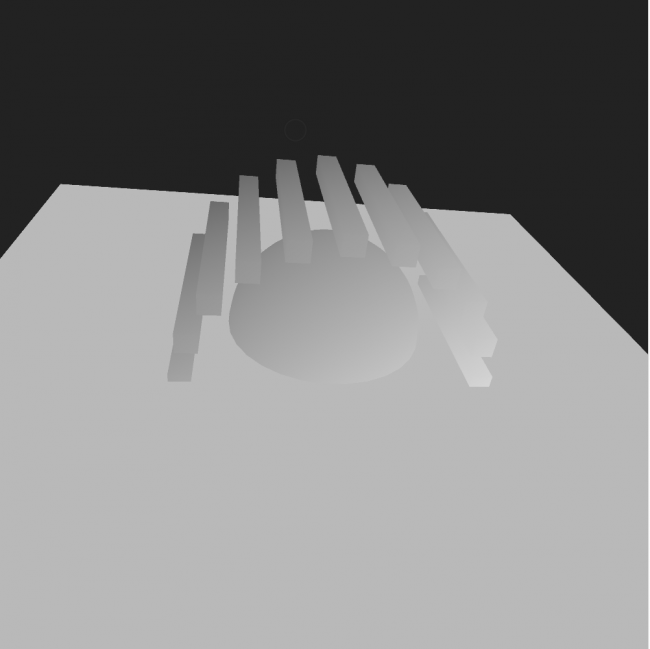`gl_FragColor = vec4( vec3(shadowCoordProj.z), 1.);`

• @kosowski: That's happening because Processing is actually using different transformation matrices for shapes (another Processing "thing").

Replace the defaultShader's vertex code with the following snipped to take that into account:

``````String[] vertSource = {
"uniform vec3 lightDirection;",
"uniform mat4 transform;",
"uniform mat4 modelview;",
"uniform mat3 normalMatrix;",

"attribute vec4 vertex;",
"attribute vec4 color;",
"attribute vec3 normal;",

"varying vec4 vertColor;",
"varying float lightIntensity;",

"void main() {",
"vertColor = color;",
"vec3 vertNormal = normalize(normalMatrix * normal);",
"vec4 vertPosition = modelview * vertex;",
"lightIntensity = 0.5 + dot(-lightDirection, vertNormal) * 0.5;",
"gl_Position = transform * vertex;",
"}"
};
``````

I'll be away for an hour or so, I'll update my shadow mapping example when I'm back.

• Updated the code:

• Added support for transformed normals (scale for example will scale both the vertex and the normal data).

PShapeOBJ example: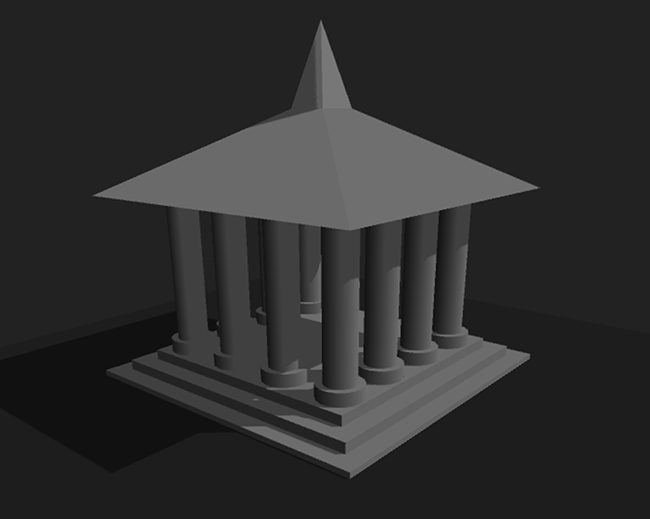• Hi, I found this code while searching for sketches in Processing that deal with shadowmapping. I found it very useful and therefore implemented it in my own program which is part of my exam (of course with mentioning the source). For my exam I need to be able to explain the implementation of this code too. Since it seems to be dealing with transformations, matrices and so on I do not really understand the consistency between al those. Could you maybe give a brief explanation about the basic idea behind shadowmapping? Thanks!

• Hey, glad you could use my code snippet and sorry for the late reply - work is killing me! :D

Atm. I basically don't have the time to do anything not work related, but this learnopengl.com article and this youtube video are doing a pretty good job at explaning how the basic algorithm works.

Hope I could help.

• Hi, no problem! Thank you for the link, very useful! Lots of succes with your work further and once again thanks a lot for saving my exam! ;)

• cool work!

• hello Poersch, I was giving a look to your good and simple implementation of projected shadows.

I'm planning to use it along with a custom displacement and coloring shader. What would be the best way to put together the two?

Do I need to blend together the "defaultShader" and my "displacementShader", or there it is a way to keep the two separated?

Thanks a lot.

• this was very helpful I at first got some errors with shaders versions and attributes .. I made it with version 410, changing varying and attributes to in and out. I will show you what I am doing .. also I want to use another shader that I currently have, what I am currently doing is rendering some PShape rectangles in 3d space and 2 lights at different positions. I am using Chromatic abbreation and vignette .. I render whole scene in PGraphics and then using it as texture to shader and then rendering it with image(pg,0,0,width,height). I will read your code and try to figure out how I use your code for shadows.

• Hi, this looks awesome and is just the thing I'm looking for.

Unfortunately, the code doesn't work for me. I get "implicit version number 110 not supported by gl3 forward compatible context", which seems weird because I see that another version number is set fragSource. I found via google that "330 core" should be set, but using this tip I still get the same error message. I'm a newbie to ogl programming in processing so I'm lost here. What can I do to fix the problem? Thanks in advance for your time and effort.

• Hello all,

I redid some work to make it work on recent mac machine, here is the code :

first processing (not much change here)

``````    import peasy.*;

PVector lightDir = new PVector();
int landscape = 1;

void setup() {
size(800, 800, P3D);
new PeasyCam(this, 300).rotateX(4.0);
initDefaultPass();
}

void draw() {

// Calculate the light direction (actually scaled by negative distance)
float lightAngle = frameCount * 0.002;
lightDir.set(sin(lightAngle) * 160, 160, cos(lightAngle) * 160);

shadowMap.camera(lightDir.x, lightDir.y, lightDir.z, 0, 0, 0, 0, 1, 0);
shadowMap.background(0xffffffff); // Will set the depth to 1.0 (maximum depth)

// Update the shadow transformation matrix and send it, the light

// Render default pass
background(0xff222222);
renderLandscape(g);

// Render light source
pushMatrix();
fill(0xffffffff);
translate(lightDir.x, lightDir.y, lightDir.z);
box(5);
popMatrix();

}

shadowMap.ortho(-200, 200, -200, 200, 10, 400); // Setup orthogonal view matrix for the directional light
}

public void initDefaultPass() {

noStroke();
perspective(60 * DEG_TO_RAD, (float)width / height, 10, 1000);
}

// Bias matrix to move homogeneous shadowCoords into the UV texture space
0.5, 0.0, 0.0, 0.5,
0.0, 0.5, 0.0, 0.5,
0.0, 0.0, 0.5, 0.5,
0.0, 0.0, 0.0, 1.0
);

// Apply project modelview matrix from the shadow pass (light direction)

// Apply the inverted modelview matrix from the default pass to get the original vertex
// positions inside the shader. This is needed because Processing is pre-multiplying
// the vertices by the modelview matrix (for better performance).
PMatrix3D modelviewInv = ((PGraphicsOpenGL)g).modelviewInv;

// Convert column-minor PMatrix to column-major GLMatrix and send it to the shader.
// PShader.set(String, PMatrix3D) doesn't convert the matrix for some reason.
));

// Calculate light direction normal, which is the transpose of the inverse of the
// modelview matrix and send it to the default shader.
float lightNormalX = lightDir.x * modelviewInv.m00 + lightDir.y * modelviewInv.m10 + lightDir.z * modelviewInv.m20;
float lightNormalY = lightDir.x * modelviewInv.m01 + lightDir.y * modelviewInv.m11 + lightDir.z * modelviewInv.m21;
float lightNormalZ = lightDir.x * modelviewInv.m02 + lightDir.y * modelviewInv.m12 + lightDir.z * modelviewInv.m22;
float normalLength = sqrt(lightNormalX * lightNormalX + lightNormalY * lightNormalY + lightNormalZ * lightNormalZ);
defaultShader.set("lightDirection", lightNormalX / -normalLength, lightNormalY / -normalLength, lightNormalZ / -normalLength);

}

public void keyPressed() {
if(key != CODED) {
if(key >= '1' && key <= '3')
landscape = key - '0';
else if(key == 'd') {
} else if(key == 's') {
}
}
}

public void renderLandscape(PGraphics canvas) {
switch(landscape) {
case 1: {
float offset = -frameCount * 0.01;
canvas.fill(0xffff5500);
for(int z = -5; z < 6; ++z)
for(int x = -5; x < 6; ++x) {
canvas.pushMatrix();
canvas.translate(x * 12, sin(offset + x) * 20 + cos(offset + z) * 20, z * 12);
canvas.box(10, 100, 10);
canvas.popMatrix();
}
} break;
case 2: {
float angle = -frameCount * 0.0015, rotation = TWO_PI / 20;
canvas.fill(0xffff5500);
for(int n = 0; n < 20; ++n, angle += rotation) {
canvas.pushMatrix();
canvas.translate(sin(angle) * 70, cos(angle * 4) * 10, cos(angle) * 70);
canvas.box(10, 100, 10);
canvas.popMatrix();
}
canvas.fill(0xff0055ff);
canvas.sphere(50);
} break;
case 3: {
float angle = -frameCount * 0.0015, rotation = TWO_PI / 20;
canvas.fill(0xffff5500);
for(int n = 0; n < 20; ++n, angle += rotation) {
canvas.pushMatrix();
canvas.translate(sin(angle) * 70, cos(angle) * 70, 0);
canvas.box(10, 10, 100);
canvas.popMatrix();
}
canvas.fill(0xff00ff55);
canvas.sphere(50);
}
}
canvas.fill(0xff222222);
canvas.box(360, 5, 360);
}
``````

``````uniform mat4 transform;
attribute vec4 vertex;
void main() {
gl_Position = transform * vertex;
}
``````

``````// In the default shader we won't be able to access the shadowMap's depth anymore,
// just the color, so this function will pack the 16bit depth float into the first
// two 8bit channels of the rgba vector.
vec4 packDepth(float depth) {
float depthFrac = fract(depth * 255.0);
return vec4(depth - depthFrac / 255.0, depthFrac, 1.0, 1.0);
}

void main(void) {
gl_FragColor = packDepth(gl_FragCoord.z);
}
``````

vertsource.vert

``````uniform mat4 transform;
uniform mat4 modelview;
uniform mat3 normalMatrix;
uniform vec3 lightDirection;
attribute vec4 vertex;
attribute vec4 color;
attribute vec3 normal;
varying vec4 vertColor;
varying float lightIntensity;

void main() {
vertColor = color;
vec4 vertPosition = modelview * vertex;// Get vertex position in model view space
vec3 vertNormal = normalize(normalMatrix * normal);// Get normal direction in model view space
lightIntensity = 0.5 + dot(-lightDirection, vertNormal) * 0.5;
gl_Position = transform * vertex;
}
``````

and finally fragsource.frag

``````#version 150

// Used a bigger poisson disk kernel than in the tutorial to get smoother results
const vec2 poissonDisk = vec2[] (
vec2(0.95581, -0.18159), vec2(0.50147, -0.35807), vec2(0.69607, 0.35559),
vec2(-0.0036825, -0.59150), vec2(0.15930, 0.089750), vec2(-0.65031, 0.058189),
vec2(0.11915, 0.78449), vec2(-0.34296, 0.51575), vec2(-0.60380, -0.41527)
);

// Unpack the 16bit depth float from the first two 8bit channels of the rgba vector
float unpackDepth(vec4 color) {
return color.r + color.g / 255.0;
}
in vec4 vertColor;
in float lightIntensity;

out vec4 FragColor;

void main(void) {

// Project shadow coords, needed for a perspective light matrix (spotlight)
vec4 col;
// Only render shadow if fragment is facing the light
if(lightIntensity > 0.5) {
float visibility = 9.0;

// I used step() instead of branching, should be much faster this way
for(int n = 0; n < 9; ++n){
}

col = vec4(vertColor.rgb * min(visibility * 0.05556, lightIntensity), vertColor.a);
} else {
col = vec4(vertColor.rgb * lightIntensity, vertColor.a);
}

FragColor = col;

}
``````

Cheers !

• Thxs for sharing!

@nabr

Kf

• edited February 2018

@berenger yeah, nice, lot of guys requested it. and finally someone found time.

kind of inconsequent you converted only 2 shaders in #version 150, what with the other two ? : ) gl_FragColor

since you are using modern version of shaders you can also use build in functions to pack unpack depth. yes, it was kind of painful 10years ago.

https://www.khronos.org/registry/OpenGL-Refpages/gl4/html/intBitsToFloat.xhtml

• Honestly yes it could be reworked :)

I only followed the path of errors processing for mac has thrown at me and correcting them one by one by iteration until it worked. So what I changed is only what was needed for it to work based on the original code.